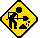## Sharp EL-512II

Datasheet legend
Ab/c: Fractions calculation
AC: Alternating current
BaseN: Number base calculations
Card: Magnetic card storage
Cmem: Continuous memory
Cond: Conditional execution
Const: Scientific constants
Cplx: Complex number arithmetic
DC: Direct current
Eqlib: Equation library
Exp: Exponential/logarithmic functions
Fin: Financial functions
Grph: Graphing capability
Hyp: Hyperbolic functions
Intg: Numerical integration
Jump: Unconditional jump (GOTO)
Lbl: Program labels
LCD: Liquid Crystal Display
LED: Light-Emitting Diode
Li-ion: Lithium-ion rechargeable battery
Lreg: Linear regression (2-variable statistics)
mA: Milliamperes of current
Mtrx: Matrix support
NiMH: Nickel-metal-hydrite rechargeable battery
Prnt: Printer
RTC: Real-time clock
Sdev: Standard deviation (1-variable statistics)
Solv: Equation solver
Subr: Subroutine call capability
Symb: Symbolic computing
Tape: Magnetic tape storage
Trig: Trigonometric functions
Units: Unit conversions
VAC: Volts AC
VDC: Volts DC
 Years of production: Display type: Numeric display New price: Display color: Black Display technology: Liquid crystal display Size: 5"×2½"×½" Display size: 10+2 digits Weight: 3 oz Entry method: Formula entry Batteries: 1×"CR-2016" Lithium Advanced functions: Trig Exp Hyp Lreg Cmem External power: Memory functions: +/- I/O: Programming model: Formula programming Precision: 12 digits Program functions: Jump Cond Memories: 13 numbers Program display: Formula display Program memory: 256 program steps Program editing: Formula entry Chipset: Forensic result:The EL-512II is an obvious predecessor to another calculator that I've had in my collection for some time, the EL-512H. Functionality-wise, the two calculators appear identical.The Gamma function:

f(X)=
1⇒G
$\hookrightarrow$X>0•N→[
G÷X⇒G
X+1⇒X
$\Lsh$]
G√2π÷X×
(1+76.18009173÷(X+1)–86.50532033÷(X+2)+
24.01409824÷(X+3)–1.23173957÷(X+4)+
1.20865E-3÷(X+5)–5.4E-6÷(X+6))
(X+5.5)Yx(X+.5)×ex-(X+5.5)